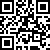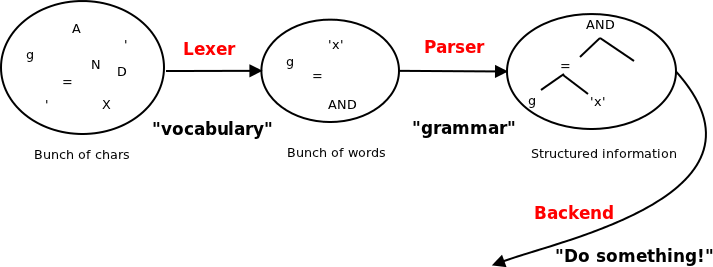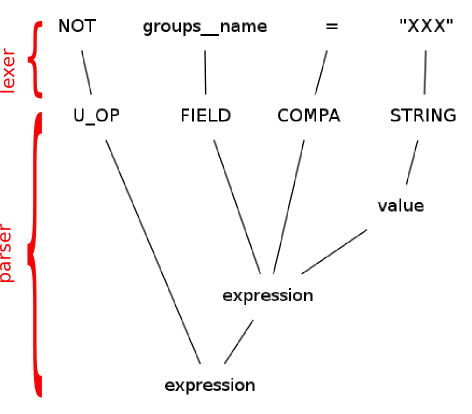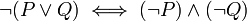# Implementing Domain-Specific Languages in Django Applications# Why would you do that? Aren't DSLs sooo 20th Century?# Initial motivation: Searching Contacts

```class Contact(models.Model):
first_name = models.CharField(max_length=50)
last_name = models.CharField(max_length=50)
#[...]
state = models.ForeignKey('State')
groups = models.ManyToManyField('Group', verbose_name=u'groupes')
```
• Groups have informal semantics
• Client wants to be able to check things like

• Everybody both in group X and Y should not be in group Z
• All contacts not in group X should have state Y
• These checks will evolve in time (no hard-coding)# Some notions are intrinsically hard to represent in GUIs

• What's the GUI for

``````pictures with width > height and not marked
rotate 90

if height > 600px
resize to height=600px

while face_detected: f
blur f
``````
• GUI multi-criteria searches are often limited to all-ANDs (sometimes all-ORs)

... but `(cond1 OR NOT cond2) AND cond3` is usually unavailable

• GUIs for actions are usually limited to a linear flow of actions# The True Reasons (but don't tell the client)

• Quick and easy to implement (if you use the right tools)
• Fun to code!# OK, it might not be for the average end-user, but...

• Some users might be power users
• Your DSL could be used as a scripting language
• The end user would only see "add-ons"# How?# OK, then. What do we need for a DSL?

• At the very least: a lexer and a parser, and some kind of backend• The lexer and parser part are quite generic
• use code generator# Introducing PLY

• PLY is an implementation of lex and yacc for Python
• http://www.dabeaz.com/ply/
• Naming conventions and introspection ⇒ very "economic" code!

Let's use it to compile expressions like

``````groups__name="XXX" AND NOT groups__name="YYY"
(modified > 1/4/2011 OR NOT state__name="OK") AND groups__name=="XXX"
``````

into `django.db.models.Q` objects# Lexer

```import ply.lex as lex

tokens = (
'COMPA',    # comparison operator
'STRING',
'NUMBER',
#[...]
)

t_COMPA = r'=|[<>]=?|~~?'

literals = '()' # shortcut for 1-char tokens

def t_STRING(t):
r'"[^"]*"'
t.value = t.value[1:-1]
return t

def t_NUMBER(t):
r'\d+'
t.value = int(t.value)
return t

# [...]

def t_error(t):
raise CompileException(u"Cannot make sense of char: %s" % t.value)
```# Parser - The Grammar

``````expression : expression B_OP expression
expression : U_OP expression
expression : '(' expression ')'
expression : FIELD COMPA value
value : STRING
| NUMBER
| DATE
``````# Parser in PLY

• Grammar rules go into docstrings
• Special argument p corresponds to rule parts

```def p_expression_u_op(p):
'''expression : U_OP expression'''
if p == 'NOT':
p = ~ p
```

means

If you encounter U_OP followed by an expression, consider this as a new expression with value determined as follows:

if the value of the U_OP is 'NOT', then the value of the final expression is the negation of the value of the initial expression# Parser in PLY - 2

```import ply.yacc as yacc

def p_value(p):
'''value : STRING
| NUMBER
| DATE'''
p = p

def p_expression_paren(p):
"expression : '(' expression ')' "
p = p

def p_expression_b_op(p):
'''expression : expression B_OP expression'''
if p == 'AND':
p = p & p
elif p == 'OR':
p = p | p
```# Parser in PLY - 3

```from django.db.models import Q

compa2lookup = {
'=': 'exact',
'~': 'contains',
'~~': 'regex',
'>': 'gt',
'>=': 'gte',
'<': 'lt',
'<=': 'lte',
}

def p_expression_ID(p):
'expression : FIELD COMPA value'

# Let's map 'groups__name = "XXX"' to
# Q(groups__name__exact="XXX")

lookup = compa2lookup[p]

field = '%s__%s' % (p, lookup)

d = {field: p}

p = Q(**d)
```# Putting it all together

```def compile(expr):
# create separate lexer and parser for each compilation
lexer = lex.lex()
parser = yacc.yacc()
# now, parse!
return parser.parse(expr,lexer=lexer)
```

This will return a Q object with corresponding query (or raise a CompileException). Just use it in your views or forms:

```# try with things like
# expr = 'groups__name="XXX" AND NOT groups__name="YYY"'
# or expr = 'modified > 1/4/2011 OR NOT state__name="OK"'

try:
q = compile(expr)
except CompileException, e:
# error handling

qs = Contact.objects.filter(q)
```# Limitations# Defeating De Morgan's Laws

• We all know that• But...

• `NOT (groups__name="XXX" OR groups__name="YYY")`

yields many more results than

• `NOT groups__name="XXX" AND NOT groups__name="YYY"`
• Is our compiler buggy?# The Dark Side of Q-objects

When making complex queries spanning multi-valued relationships

• If you `AND` 2 Q-objects, the conditions can apply to different values of the relationship

```a = Q(groups__name="XXX")
b = Q(groups__name="YYY")

q1 = (~a) & (~b)
# yields same results as
list(Contact.objects.exclude(groups__name="XXX").exclude(groups__name="YYY"))
```
• If you `OR` 2 Q-objects, the conditions must apply to the same value of the relationship

```q2 = ~ (a | b)
# yields same results as
result = []
for c in Contact.objects.all():
for g in c.groups.all():
if not g.name in ["XXX","YYY"]:
result.append(c)
```
• Not really documented in Django's doc# Other limitations

• Limited to Django's ORM functionalities
• Only a query language (as opposed to scripting language)# Going Further# Functional approach

• Instead of (or in addition to) building Q-objects, you can build functions

```def p_statement(p):
'statement : ACTION expression'

if p == 'MARK':
p = lambda: Contact.objects.filter(p).update(marked=True)
elif ...
```
• Now `compile` returns a function

```f = compile('MARK groups__name="XXX"')
# Now, execute the action
f()
```# Save the earth, grow a tree

If you need something substantially more complicated, you will probably need to

• compile to a tree structure

```def p_expression_op(p):
p = OpNode(op=p, children=[p, p])
```
• write an interpreter, e.g. a recursive one:

```class OpNode:

def execute(self):
args = [c.execute() for c in self.children]
if self.op == '+':